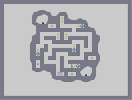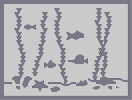### It's GUESS THAT HOTTIE TIME!Hover over the thumbnail for a full-size version.

Author BE_nSPIRED art author:be_nspired face hottie n-art rated 2010-01-06 2010-01-06 5 by 10 people. \$It's GUESS THAT HOTTIE TIME!#BE_nSPIRED#n-art#00000000000000000000000000000000000000000000000000000000000000000000000000000000000000000000000000000000000000000000000000000000000000000000000000000000000000000000000000000000000000000000000000000000000000000000000000000000000000000000000000000000000000000000000000000000000000000000000000000000000000000000000000000000000000000000000000000000000000000000000000000000000000000000000000000000000000000000000000000000000000000000000000000000000000000000000000000000000000000000000000000000000000000000000000000000000000000000000000000000000000000000000000000000000000000000000000000000000000000000000000000000000000000000000000000000000000000000000000000000000000000000000000000000000000000000000000000000000000000|10^456,354!10^459,354!10^460,352!10^463,352!10^465,348!10^466,346!10^467,344!10^469,344!10^470,342!10^471,340!10^474,337!10^475,337!10^475,335!10^477,335!10^478,333!10^479,331!10^479,329!10^482,327!10^484,323!10^486,323!10^487,323!10^489,319!10^490,317!10^492,315!10^494,313!10^494,313!10^496,310!10^498,308!10^501,304!10^502,302!10^504,302!10^504,300!10^506,300!10^508,298!10^510,296!10^513,292!10^516,288!10^516,286!10^516,284!10^509,285!10^509,285!10^507,283!10^505,280!10^505,274!10^505,266!10^506,264!10^502,266!10^497,266!10^490,267!10^483,262!10^487,253!10^488,250!10^488,258!10^488,258!10^488,246!10^488,240!10^484,229!10^484,216!10^480,228!10^474,235!10^467,240!10^464,244!10^467,233!10^467,229!10^462,241!10^453,251!10^452,243!10^454,228!10^455,235!10^447,248!10^445,245!10^446,230!10^446,230!10^440,233!10^423,236!10^429,228!10^429,230!10^423,234!10^401,245!10^406,248!10^421,235!10^419,236!10^409,244!10^403,248!10^392,252!10^398,243!10^400,238!10^392,248!10^383,254!10^380,257!10^387,243!10^392,230!10^394,216!10^394,208!10^394,208!10^395,230!10^388,239!10^378,255!10^378,255!10^373,257!10^374,253!10^378,239!10^382,228!10^383,217!10^371,239!10^364,247!10^358,254!10^353,260!10^358,245!10^347,250!10^346,251!10^345,258!10^337,260!10^331,262!10^328,262!10^333,249!10^326,257!10^326,260!10^326,262!10^321,262!10^313,257!10^306,251!10^300,251!10^295,249!10^275,249!10^306,255!10^281,249!10^278,251!10^264,255!10^264,252!10^254,247!10^254,240!10^249,232!10^249,227!10^245,225!10^243,217!10^243,210!10^245,204!10^452,358!10^452,360!10^452,362!10^453,366!10^453,367!10^453,367!10^453,368!10^453,353!10^448,350!10^448,348!10^445,345!10^437,341!10^434,341!10^430,337!10^426,337!10^420,337!10^418,337!10^412,337!10^407,337!10^402,337!10^400,337!10^394,337!10^390,338!10^383,339!10^379,339!10^375,339!10^368,340!10^363,341!10^357,342!10^348,343!10^346,343!10^341,344!10^340,348!10^337,349!10^334,349!10^330,349!10^327,347!10^340,353!10^341,355!10^343,357!10^345,361!10^347,363!10^348,367!10^351,371!10^353,372!10^359,376!10^361,378!10^362,379!10^368,379!10^369,380!10^450,373!10^448,374!10^446,377!10^443,379!10^442,381!10^435,383!10^431,385!10^426,385!10^424,385!10^416,385!10^413,386!10^409,386!10^404,386!10^400,387!10^394,387!10^388,387!10^388,387!10^384,387!10^381,387!10^378,385!10^377,385!10^374,383!10^373,383!10^371,383!10^367,381!10^359,379!10^323,347!10^321,348!10^314,348!10^312,346!10^308,344!10^306,342!10^304,342!10^301,340!10^296,336!10^293,336!10^290,336!10^286,336!10^274,332!10^269,331!10^267,331!10^280,334!10^259,330!10^253,329!10^249,329!10^245,329!10^239,329!10^237,329!10^232,330!10^228,336!10^228,339!10^229,342!10^231,345!10^234,349!10^235,352!10^237,353!10^239,353!10^243,359!10^245,362!10^247,364!10^262,371!10^252,368!10^263,375!10^267,376!10^271,377!10^276,378!10^283,378!10^287,379!10^291,380!10^291,380!10^298,380!10^317,350!10^317,351!10^317,355!10^317,359!10^317,362!10^317,367!10^314,370!10^311,373!10^311,374!10^306,379!10^299,381!10^294,381!10^287,381!10^282,381!10^273,377!10^268,375!10^260,375!10^255,370!10^252,369!10^248,365!10^234,324!10^262,293!10^264,291!10^446,279!10^446,279!10^442,275!10^440,272!10^435,272!10^428,272!10^424,272!10^420,272!10^417,274!10^414,275!10^411,276!10^409,277!10^404,278!10^402,279!10^398,279!10^395,279!10^391,279!10^387,280!10^385,282!10^382,283!10^380,286!10^377,287!10^302,285!10^299,284!10^295,284!10^288,282!10^286,282!10^281,280!10^278,280!10^275,282!10^272,283!10^245,202!10^245,200!10^247,195!10^249,191!10^251,186!10^251,178!10^251,176!10^255,169!10^257,163!10^257,161!10^258,160!10^262,150!10^263,150!10^265,144!10^267,138!10^269,134!10^272,129!10^273,124!10^277,121!10^281,114!10^284,111!10^287,108!10^291,100!10^293,98!10^295,93!10^298,91!10^304,87!10^308,83!10^314,79!10^316,79!10^324,74!10^329,72!10^331,70!10^333,70!10^337,68!10^342,66!10^348,62!10^348,62!10^352,59!10^358,55!10^361,53!10^364,53!10^380,51!10^374,50!10^376,50!10^385,50!10^390,48!10^394,44!10^400,44!10^406,44!10^407,44!10^417,44!10^418,44!10^427,46!10^429,47!10^438,47!10^443,47!10^447,47!10^455,47!10^455,47!10^462,47!10^469,48!10^469,49!10^481,49!10^482,51!10^483,51!10^490,60!10^493,62!10^510,62!10^492,61!10^506,65!10^509,65!10^508,65!10^499,66!10^514,73!10^515,73!10^526,74!10^530,77!10^524,71!10^503,67!10^477,58!10^473,51!10^465,47!10^457,50!10^444,51!10^411,47!10^409,47!10^400,47!10^512,298!10^515,298!10^515,304!10^517,310!10^519,312!10^525,306!10^527,304!10^537,293!10^538,291!10^543,294!10^543,292!10^544,289!10^550,281!10^552,279!10^555,277!10^559,272!10^562,270!10^567,266!10^569,260!10^573,254!10^573,251!10^573,245!10^574,242!10^575,237!10^576,232!10^577,227!10^577,226!10^579,216!10^579,215!10^577,211!10^577,205!10^578,200!10^578,199!10^578,197!10^578,190!10^576,186!10^576,178!10^576,177!10^574,168!10^572,164!10^572,159!10^572,152!10^572,151!10^565,144!10^566,139!10^566,137!10^564,134!10^560,128!10^558,125!10^556,123!10^556,119!10^553,113!10^553,109!10^547,104!10^542,99!10^538,94!10^534,90!10^530,83!10^530,80!10^446,311!10^442,307!10^440,305!10^437,303!10^430,299!10^427,297!10^425,297!10^418,297!10^414,297!10^409,297!10^401,299!10^396,300!10^391,301!10^381,308!10^381,309!10^378,310!10^376,312!10^374,313!10^385,304!10^445,316!10^438,319!10^434,321!3^428,323!3^381,317!3^391,319!3^401,323!3^414,324!10^408,307!10^404,308!10^404,311!10^407,315!10^411,316!10^415,316!10^415,311!10^415,306!10^410,306!10^407,308!10^405,313!10^409,317!10^414,318!10^419,314!10^415,306!10^409,304!10^405,304!10^406,310!10^411,313!10^417,309!10^417,309!10^417,311!10^413,312!10^322,308!10^320,308!10^318,306!10^317,306!10^312,304!10^308,300!10^305,300!10^296,298!10^293,298!10^290,299!10^288,300!10^284,302!10^284,302!10^304,310!10^306,312!10^309,313!10^310,313!10^313,313!10^310,311!10^308,311!3^325,314!3^315,317!3^309,316!3^299,317!3^290,313!3^306,319!3^263,259!3^264,262!3^264,266!3^264,273!3^265,280!3^268,289!3^268,292!3^268,299!3^268,306!3^266,308!3^266,318!3^271,325!3^271,325!3^274,342!3^274,345!3^274,352!3^274,355!3^276,361!3^278,378!3^278,380!3^282,385!3^275,367!3^279,374!3^282,380!3^283,387!3^285,397!3^289,401!3^290,403!3^294,408!3^295,409!3^298,411!3^299,414!3^303,421!3^304,422!3^307,425!3^309,432!3^310,434!3^311,437!3^314,442!3^315,446!3^321,449!3^324,454!3^326,458!3^328,461!3^331,465!3^331,466!3^333,469!3^335,474!3^342,481!3^343,488!3^353,502!3^354,503!3^348,492!3^348,494!3^357,508!3^357,509!3^369,522!3^371,522!3^363,517!3^362,514!3^366,519!3^374,523!3^376,523!3^382,525!3^390,527!3^391,527!3^392,527!3^404,527!3^407,527!3^413,527!3^416,525!3^420,521!3^422,521!3^436,521!3^432,522!3^437,520!3^451,512!3^452,512!3^442,516!3^442,516!3^449,514!3^460,508!3^462,506!3^466,500!3^469,498!3^477,494!3^480,492!3^484,489!3^488,485!3^490,480!3^494,475!3^498,469!3^500,465!3^501,463!3^502,458!3^562,279!3^566,277!3^572,276!3^572,280!3^572,287!3^573,292!3^573,296!3^573,303!3^569,307!3^565,311!3^565,313!3^565,315!3^561,322!3^557,328!3^557,330!3^557,333!3^557,334!3^557,337!3^557,337!3^527,361!3^531,359!3^533,356!3^538,352!3^540,350!3^545,346!3^555,335!3^553,337!3^551,338!3^546,341!3^541,345!3^528,327!3^531,327!3^535,320!3^538,316!3^543,308!3^545,301!3^546,312!3^549,312!3^543,312!3^543,313!3^524,352!3^525,357!3^525,362!3^525,369!3^525,374!3^525,377!3^525,383!3^525,389!3^523,395!3^523,406!3^524,416!3^525,419!3^526,422!3^529,427!3^531,429!3^534,433!3^536,438!3^537,443!3^541,447!3^544,450!3^547,452!3^548,452!3^553,452!3^553,456!3^330,316!3^330,320!3^328,325!3^326,330!3^324,338!3^324,345!3^317,376!3^315,384!3^313,391!3^313,395!3^313,402!3^315,409!3^318,412!3^322,414!3^326,414!3^333,414!3^336,410!3^342,408!3^351,408!3^358,411!3^362,411!3^370,411!3^378,409!3^381,405!3^267,291!3^267,294!3^267,291!3^267,287!3^263,283!3^262,282!3^262,276!3^266,303!3^267,306!3^267,312!3^267,315!10^237,322!10^243,316!10^248,314!10^249,312!10^251,312!10^253,310!10^255,310!10^257,306!10^258,306!10^239,321!10^425,430!10^425,431!10^423,433!10^419,439!10^419,440!10^417,442!10^413,445!10^409,447!10^407,451!10^405,455!10^401,458!10^398,460!10^392,462!10^389,463!10^383,464!10^378,465!10^374,465!10^371,465!10^367,465!10^363,463!10^357,457!10^356,455!10^353,455!10^351,455!10^351,453!10^349,452!3^414,430!3^411,431!3^408,432!3^407,434!3^400,437!3^395,439!3^392,440!3^389,441!3^384,442!3^379,443!3^373,443!3^371,441!3^369,441!3^364,441!3^360,441!3^356,439!3^351,437!3^350,437!3^347,437!3^342,437!3^340,437!3^340,439!3^345,442!3^347,445!3^352,452!3^358,450!3^365,450!3^370,452!3^373,452!3^385,450!3^393,448!3^401,444!3^389,451!3^382,450!3^565,460!3^577,468!3^586,473!3^600,478!3^620,481!3^637,490!3^651,500!3^677,501!3^706,510!3^730,525!3^757,542!3^559,464!3^557,471!3^553,482!3^551,492!3^543,507!3^524,521!3^503,537!3^477,550!3^415,566!3^317,456!3^317,459!3^313,462!3^303,466!3^297,468!3^290,471!3^279,475!3^273,477!3^248,487!3^232,493!3^202,500!3^162,522!3^127,534!3^84,558!3^368,551!10^336,78!10^323,84!10^318,87!10^310,92!10^302,97!10^295,105!10^288,113!10^285,120!10^275,130!10^271,144!10^263,161!10^255,189!10^255,209!10^255,222!10^257,206!10^259,199!10^262,189!10^263,179!10^266,170!10^270,159!10^272,154!10^280,137!10^284,128!10^290,122!10^298,110!10^302,105!10^313,97!10^324,89!10^332,78!10^351,70!10^345,75!10^327,93!10^319,98!10^305,112!10^297,120!10^288,133!10^283,147!10^281,159!10^277,180!10^269,199!10^266,214!10^266,231!10^266,239!10^266,239!10^273,240!10^276,236!10^276,228!10^276,219!10^276,208!10^279,194!10^280,173!10^280,169!10^289,154!10^294,146!10^302,131!10^308,122!10^316,112!10^324,102!10^340,85!10^344,80!10^356,71!10^361,66!10^369,60!10^286,237!10^288,237!10^288,219!10^290,207!10^290,203!10^290,190!10^291,178!10^294,167!10^303,152!10^311,139!10^312,136!10^319,124!10^327,114!10^332,107!10^342,97!10^351,92!10^353,90!10^360,84!10^365,77!10^373,69!10^380,65!10^387,59!10^389,59!10^303,242!10^303,240!10^303,226!10^303,223!10^303,214!10^303,202!10^305,192!10^305,192!10^315,171!10^315,168!10^302,176!10^305,180!10^310,165!10^317,151!10^321,148!10^311,166!10^320,150!10^327,137!10^334,130!10^338,123!10^342,118!10^347,111!10^356,103!10^365,94!10^371,89!10^378,83!10^381,79!10^385,74!10^392,66!10^398,60!10^407,51!10^319,249!10^320,245!10^314,236!10^315,230!10^317,224!10^318,219!10^318,212!10^318,209!10^319,195!10^321,189!10^322,184!10^325,177!10^327,172!10^330,165!10^332,162!10^335,153!10^337,152!10^343,141!10^347,137!10^355,129!10^362,125!10^367,117!10^370,114!10^374,108!10^381,103!10^387,94!10^393,90!10^399,83!10^408,72!10^414,58!10^388,89!10^367,109!10^367,112!10^364,107!10^355,116!10^349,121!10^342,128!10^332,150!10^311,194!10^307,220!10^330,242!10^331,234!10^331,233!10^330,228!10^332,217!10^332,216!10^332,207!10^334,194!10^337,183!10^340,175!10^342,175!10^346,167!10^351,161!10^354,155!10^356,150!10^362,141!10^370,133!10^376,128!10^382,119!10^386,112!10^389,108!10^393,102!10^401,93!10^408,84!10^413,78!10^420,62!10^407,62!10^398,76!10^377,90!10^364,111!10^347,146!10^348,157!10^342,252!10^346,244!10^344,235!10^341,229!10^341,223!10^344,213!10^345,206!10^345,195!10^348,191!10^353,182!10^356,176!10^360,168!10^363,163!10^370,155!10^378,147!10^381,141!10^387,133!10^395,122!10^399,114!10^408,100!10^411,97!10^418,85!10^421,75!10^424,68!10^425,63!10^389,83!10^378,90!10^365,101!10^341,126!10^287,153!10^291,180!10^310,167!10^312,164!10^324,160!10^300,161!10^294,163!10^326,131!10^368,98!10^334,155!10^326,191!10^322,203!10^384,158!3^354,493!3^364,507!3^375,512!3^388,521!3^352,484!3^341,472!3^331,456!3^319,437!3^304,415!3^318,395!3^323,409!3^273,258!3^275,269!3^276,295!3^276,316!3^276,304!3^282,345!3^286,362!3^286,355!3^293,383!3^293,401!3^305,417!10^357,239!10^355,229!10^352,232!10^352,225!10^354,217!10^355,211!10^355,206!10^359,196!10^361,187!10^365,178!10^370,169!10^375,160!10^381,154!10^391,142!10^391,139!10^401,129!10^401,126!10^408,114!10^410,111!10^414,100!10^419,92!10^424,83!10^429,72!10^428,62!10^426,55!10^431,53!10^436,56!10^442,61!10^438,65!10^437,71!10^438,77!10^434,85!10^432,90!10^428,95!10^421,101!10^421,106!10^416,119!10^412,123!10^403,140!10^399,144!10^393,157!10^390,161!10^381,169!10^376,180!10^374,190!10^368,202!10^368,222!10^369,233!10^367,231!10^367,215!10^366,210!10^256,242!10^278,222!10^288,226!10^292,228!10^294,224!10^299,210!10^304,193!10^287,202!10^276,216!10^263,226!10^269,206!10^275,187!10^289,176!10^302,180!10^306,187!10^315,184!10^315,178!10^299,164!10^314,141!10^332,121!10^313,156!10^310,158!10^304,148!10^296,171!10^296,167!10^301,137!10^303,130!10^293,138!10^293,141!10^368,220!10^380,214!10^385,198!10^378,206!10^375,208!10^386,194!10^387,189!10^389,184!10^393,180!10^395,174!10^397,170!10^399,169!10^402,162!10^405,157!10^407,155!10^409,152!10^410,148!10^414,141!10^417,138!10^419,133!10^420,130!10^427,119!10^428,115!10^430,111!10^434,104!10^441,82!10^447,69!10^451,64!10^468,58!10^462,59!10^449,72!10^464,68!10^469,66!10^457,70!10^455,74!10^446,80!10^473,76!10^493,70!10^470,75!10^446,86!10^435,95!10^466,81!10^502,81!10^491,81!10^465,91!10^454,97!10^436,110!10^453,95!10^485,82!10^476,73!10^482,72!10^473,88!10^461,98!10^444,105!10^430,121!10^433,124!10^442,115!10^447,110!10^451,106!10^467,98!10^474,96!10^497,90!10^506,90!10^516,87!10^518,85!10^524,90!10^516,96!10^508,97!10^500,97!10^493,97!10^489,97!10^483,98!10^473,103!10^467,108!10^460,115!10^454,119!10^452,122!10^444,129!10^407,235!10^407,224!10^407,215!10^402,209!10^402,203!10^402,199!10^402,191!10^403,184!10^411,173!10^413,168!10^413,168!10^425,224!10^419,222!10^419,216!10^413,208!10^414,202!10^416,196!10^417,185!10^418,167!10^423,154!10^426,147!10^434,135!10^447,130!10^472,116!10^489,105!10^514,101!10^527,99!10^536,101!10^555,123!10^549,127!10^562,151!10^560,152!10^549,136!10^540,118!10^537,116!10^529,116!10^519,114!10^515,112!10^506,112!10^493,113!10^481,117!10^469,123!10^461,129!10^459,132!10^447,146!10^445,147!10^441,148!10^441,157!10^441,159!10^437,166!10^417,197!10^421,220!10^431,218!10^428,208!10^424,200!10^424,191!10^427,183!10^430,171!10^431,160!10^439,144!10^457,136!10^507,130!10^516,126!10^539,134!10^529,129!10^504,123!10^492,128!10^483,132!10^473,137!10^467,150!10^455,162!10^449,173!10^452,195!10^454,235!10^448,223!10^444,223!10^442,212!10^442,205!10^435,196!10^436,190!10^440,182!10^446,168!10^457,147!10^465,137!10^476,120!10^488,112!10^511,110!10^533,114!10^543,126!10^565,142!10^549,148!10^539,138!10^528,137!10^519,138!10^510,138!10^503,138!10^492,141!10^479,148!10^473,156!10^468,162!10^452,178!10^457,178!10^457,182!10^457,183!10^456,190!10^470,227!10^470,225!10^455,218!10^452,213!10^453,212!10^460,202!10^466,191!10^471,183!10^466,175!10^473,168!10^483,163!10^497,155!10^498,155!10^488,155!10^505,147!10^511,149!10^551,158!10^561,163!10^539,154!10^535,152!10^526,150!10^515,153!10^505,159!10^488,170!10^485,172!10^478,177!10^475,184!10^472,198!10^470,210!10^470,215!10^492,217!10^519,284!10^526,271!10^518,271!10^518,272!10^514,258!10^510,252!10^502,255!10^500,253!10^498,247!10^498,239!10^499,231!10^497,216!10^492,207!10^485,207!10^485,204!10^485,192!10^493,172!10^500,168!10^489,190!10^493,186!10^501,174!10^536,159!10^549,173!10^569,178!10^553,170!10^540,171!10^538,171!10^519,166!10^522,164!10^512,172!10^510,184!10^508,191!10^499,198!10^501,207!10^503,220!10^509,234!10^510,243!10^511,247!10^520,262!10^532,285!10^554,282!10^534,280!10^535,278!10^541,272!10^548,267!10^553,260!10^559,256!10^561,252!10^565,241!10^565,236!10^565,225!10^565,217!10^565,212!10^565,202!10^565,195!10^559,185!10^556,183!10^551,181!10^542,179!10^528,173!10^519,178!10^520,185!10^518,194!10^516,198!10^510,204!10^510,208!10^512,213!10^513,220!10^516,229!10^518,238!10^519,240!10^521,249!10^528,261!10^532,265!10^542,258!10^548,251!10^554,245!10^552,232!10^556,217!10^557,209!10^551,202!10^551,196!10^541,187!10^537,185!10^528,193!10^526,204!10^524,209!10^524,218!10^526,238!10^529,244!10^533,244!10^540,238!10^542,231!10^543,215!10^539,199!10^534,209!10^532,218!10^535,221!10^529,230!10^549,222!10^535,242!10^641,322!10^636,326!10^632,330!10^629,332!10^622,336!10^618,338!10^616,340!10^612,341!10^608,343!10^603,347!10^599,348!10^601,348!10^603,350!10^609,352!10^611,353!10^617,356!10^619,357!10^624,359!10^632,360!10^634,361!10^641,364!10^643,366!10^645,368!10^660,316!10^664,316!10^667,318!10^673,318!10^678,320!10^684,323!10^688,326!10^690,330!10^685,337!10^682,340!10^673,342!10^666,343!10^666,343!10^673,344!10^677,345!10^686,347!10^690,351!10^690,355!10^692,356!10^692,365!10^685,370!10^678,372!10^672,372!10^664,372# Three guesses who this NUMA HOTTIE IS. <3

## Other maps by this authorDon't dance with the Drones... Kabrizzly Bear Lone Ranger Under the sea Furry Fury Unfinished White stripes

Pages: (0)

### I

love that little crinkle in the eyebrow
Harry potter? I'm honored

### I approve of this map. <3

I'd be a bit scared if it was anyone but you.

Also, would I be the kind of secretary who gets hit on all the time?

### 0

I rate this map a 0

### It actually looks like

me in year 5. O_.

### Whee.

http://n.pastebin.com/m5a7acb

### Hmm

He kinda looks like a secretary, but I don't know why.

### Damn

Beaten by 20 minutes.

### And...

O_o Yanni commented. /me looks out the window to see a Blue Moon.
DING DING DING YANNI WINS!

### IT IS

Matthew James Mc-"Life"-Naughton the 247th.
I greater than 3 you.